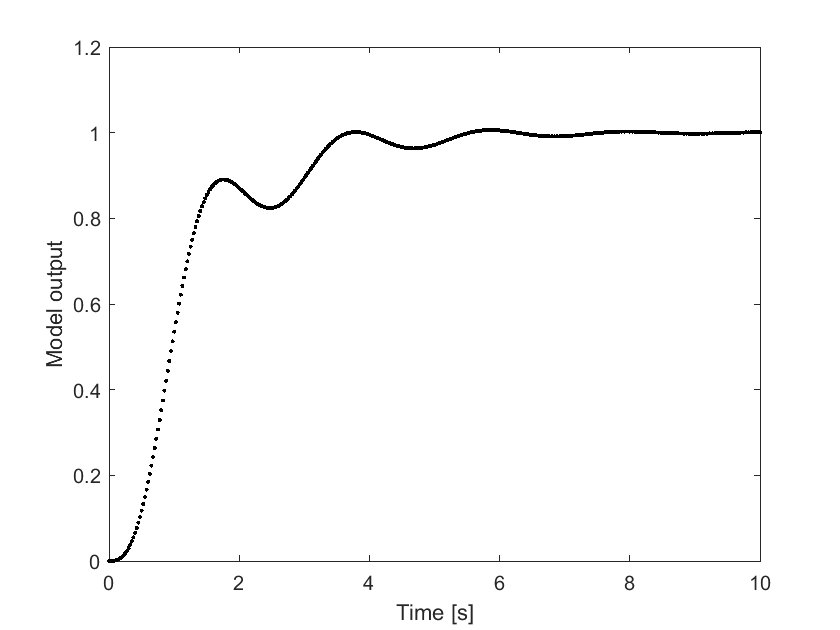# Representing transfer function as the model for Bayesian inversion problem

Dear UQ community,

I am trying to solve a Bayesian inversion problem in which a transfer function is treated as the model. The transfer function is given by \frac{8}{s^3+2s^2+10s+8}..
Following this, the model coefficients for the function can be represented by X(:,1) = 1, X(:,2) = 2, X(:,3) = 10 and X(:,4) = 8. Using this, the .mFile is written as:

function V = uq_dynamic(X)
s = tf('s');
sys_closed = (X(:,4))./((X(:,1).*(s^3))+(X(:,2).*(s^2))+(X(:,3).*(s))+(X(:,4)));
t = linspace(0,10,400);
y = step(sys_closed,t);
V = y;


In doing so, I get an error in line 3: Undefined function 'rdivide' for input arguments of type 'tf'. Is there any mistake in my vector representation of the model?

Best,
Vinit

Hi @Vinit

This is not a UQLab issue, it looks like your function uq_dynamics cannot be evaluated for a given set of parameters. I am not familiar with tf, but it looks like the operation you define in line 3 is not valid MATLAB syntax.

Before running a Bayesian inversion analysis, make sure that your forward model can be evaluated.

1 Like

Hi @Vinit,

I am not sure, but it seems to me that evaluating the MATLAB function step for several sets of parameter values with one function call may require to use a Model Array and that the construction in your post may not work in this situation. But, if you have a version of your function that can work with an input parameter like [1,2,10,8] I suggest to use this version of the function in the definition of the model and use also the flag modelopts.isVectorized = false;, following UQLab User Manual for models, Sec 2.1.1, such that UQLab is informed that your function is not vectorized. Maybe this can help, but I am not sure.

Two further remarks:

• Since multiplying all your parameter by the same factor will not change the result, you may have problems with all kinds of parameter identification, including Bayesian inversion. Therefore, I suggest to get rid of the last of your parameters.
• Since the output of the model must be of the same size as the vector for one observation: Do your really have a vector with 400 (or 401) data points with the requested function values, or do you need to extract some values from the output of the step function to get the desired output of the model?

Greetings
Olaf

2 Likes